# Surrogate Optimization of Six-Element Yagi-Uda Antenna

This example shows how to optimize an antenna design using the surrogate optimization solver. The radiation patterns of antennas depend sensitively on the parameters that define the antenna shapes. Typically, the features of a radiation pattern have multiple local optima. To calculate a radiation pattern, this example uses Antenna Toolbox™ functions.

A Yagi-Uda antenna is a widely used radiating structure for a variety of applications in commercial and military sectors. This antenna can receive TV signals in the VHF-UHF range of frequencies . The Yagi-Uda is a directional traveling-wave antenna with a single driven element, usually a folded dipole or a standard dipole, which is surrounded by several passive dipoles. The passive elements form the reflector and director. These names identify the positions relative to the driven element. The reflector dipole is behind the driven element, in the direction of the back lobe of the antenna radiation. The director dipole is in front of the driven element, in the direction where a main beam forms.

### Design Parameters

Specify the initial design parameters in the center of the VHF band .

```freq = 165e6; wirediameter = 19e-3; c = physconst("lightspeed"); lambda = c/freq;```

### Create Yagi-Uda Antenna

The driven element for the Yagi-Uda antenna is a folded dipole, a standard exciter for this type of antenna. Adjust the length and width parameters of the folded dipole. Because cylindrical structures are modeled as equivalent metal strips, calculate the width using the `cylinder2strip` utility function available in the Antenna Toolbox™. The length is $\lambda /2$ at the design frequency.

```d = dipoleFolded; d.Length = lambda/2; d.Width = cylinder2strip(wirediameter/2); d.Spacing = d.Length/60;```

Create a Yagi-Uda antenna with the exciter as the folded dipole. Set the lengths of the reflector and director elements to be $\lambda /2$. Set the number of directors to four. Specify the reflector and director spacing as $0.3\lambda$ and $0.25\lambda$, respectively. These settings provide an initial guess and serve as a starting point for the optimization procedure. Show the initial design.

```Numdirs = 4; refLength = 0.5; dirLength = 0.5*ones(1,Numdirs); refSpacing = 0.3; dirSpacing = 0.25*ones(1,Numdirs); initialdesign = [refLength dirLength refSpacing dirSpacing].*lambda; yagidesign = yagiUda; yagidesign.Exciter = d; yagidesign.NumDirectors = Numdirs; yagidesign.ReflectorLength = refLength*lambda; yagidesign.DirectorLength = dirLength.*lambda; yagidesign.ReflectorSpacing = refSpacing*lambda; yagidesign.DirectorSpacing = dirSpacing*lambda; show(yagidesign)```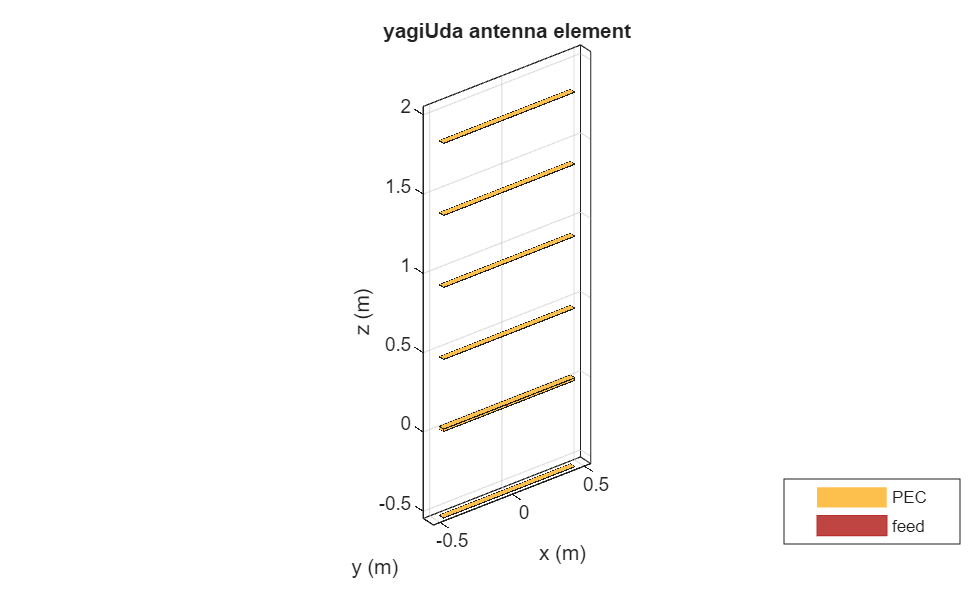### Plot Radiation Pattern at Design Frequency

Prior to executing the optimization process, plot the radiation pattern for the initial guess in 3-D.

```fig1 = figure; pattern(yagidesign,freq);```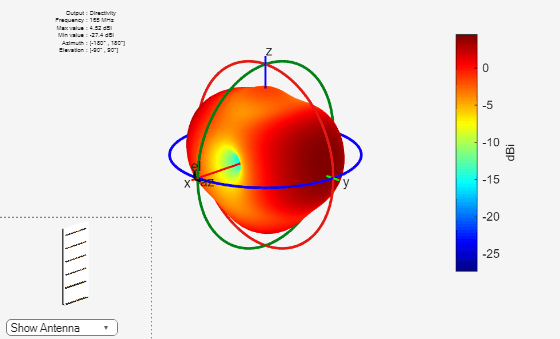This antenna does not have a higher directivity in the preferred direction, at zenith (elevation = 90 deg). This initial Yagi-Uda antenna design is a poorly designed radiator.

### Set Up Optimization

Use the following variables as control variables for the optimization:

• Reflector length (1 variable)

• Director lengths (4 variables)

• Reflector spacing (1 variable)

• Director spacings (4 variables)

In terms of a single vector parameter `parasiticVals`, use these settings:

• Reflector length = `parasiticVals(1)`

• Director lengths = `parasiticVals(2:5)`

• Reflector spacing = `parasiticVals(6)`

• Director spacings = `parasiticVals(7:10)`

In terms of `parasiticVals`, set an objective function that aims to have a large value in the 90-degree direction, a small value in the 270-degree direction, and a large value of maximum power between the elevation beamwidth angle bounds.

`type yagi_objective_function2.m`
```function objectivevalue = yagi_objective_function2(y,parasiticVals,freq,elang) % yagi_objective_function2 returns the objective for a 6-element Yagi % objective_value = yagi_objective_function(y,parasiticvals,freq,elang) % assigns the appropriate parasitic dimensions, parasiticvals, to the Yagi % antenna y, and uses the frequency freq and angle pair elang to calculate % the objective function value. % The yagi_objective_function2 function is used for an internal example. % Its behavior might change in subsequent releases, so it should not be % relied upon for programming purposes. % Copyright 2014-2018 The MathWorks, Inc. bw1 = elang(1); bw2 = elang(2); y.ReflectorLength = parasiticVals(1); y.DirectorLength = parasiticVals(2:y.NumDirectors+1); y.ReflectorSpacing = parasiticVals(y.NumDirectors+2); y.DirectorSpacing = parasiticVals(y.NumDirectors+3:end); output = calculate_objectives(y,freq,bw1,bw2); output = output.MaxDirectivity + output.FB; objectivevalue= -output; % To maximize end function output = calculate_objectives(y,freq,bw1,bw2) %calculate_objectives calculate the objective function % output = calculate_objectives(y,freq,bw1,bw2) Calculate the directivity % in az = 90 plane that covers the main beam, sidelobe and backlobe. % Calculate the maximum directivity, sidelobe level and backlobe and store % in fields of the output variable structure. [es,~,el] = pattern(y,freq,90,0:1:270); el1 = el < bw1; el2 = el > bw2; el3 = el>bw1&el<bw2; emainlobe = es(el3); esidelobes =([es(el1);es(el2)]); Dmax = max(emainlobe); SLLmax = max(esidelobes); Backlobe = es(end); F = es(91); B = es(end); F_by_B = F-B; output.MaxDirectivity= Dmax; output.MaxSLL = SLLmax; output.BackLobeLevel = Backlobe; output.FB = F_by_B; end ```

Set bounds on the control variables.

```refLengthBounds = [0.4; 0.6]; dirLengthBounds = [0.35 0.35 0.35 0.35; % lower bound on director length 0.495 0.495 0.495 0.495]; % upper bound on director length refSpacingBounds = [0.05; % lower bound on reflector spacing 0.30]; % upper bound on reflector spacing dirSpacingBounds = [0.05 0.05 0.05 0.05; % lower bound on director spacing 0.23 0.23 0.23 0.23]; % upper bound on director spacing LB = [refLengthBounds(1) dirLengthBounds(1,:) refSpacingBounds(1) dirSpacingBounds(1,:) ].*lambda; UB = [refLengthBounds(2) dirLengthBounds(2,:) refSpacingBounds(2) dirSpacingBounds(2,:) ].*lambda;```

Set the initial point for the optimization, and set the elevation beamwidth angle bounds.

```parasitic_values = [ yagidesign.ReflectorLength, ... yagidesign.DirectorLength, ... yagidesign.ReflectorSpacing ... yagidesign.DirectorSpacing]; elang = [60 120]; % elevation beamwidth angles at az = 90```

### Surrogate Optimization

To search for a global optimum of the objective function, use `surrogateopt` as the solver. Set options to allow 500 function evaluations, include the initial point, use parallel computation, and use the `'surrogateoptplot'` plot function. To understand the `'surrogateoptplot'` plot, see Interpret surrogateoptplot..

```surrogateoptions = optimoptions("surrogateopt", MaxFunctionEvaluations=500,... InitialPoints=parasitic_values, UseParallel=canUseGPU(), PlotFcn="surrogateoptplot"); rng(4) % For reproducibility optimdesign = surrogateopt(@(x) yagi_objective_function2(yagidesign,x,freq,elang),... LB,UB,surrogateoptions);```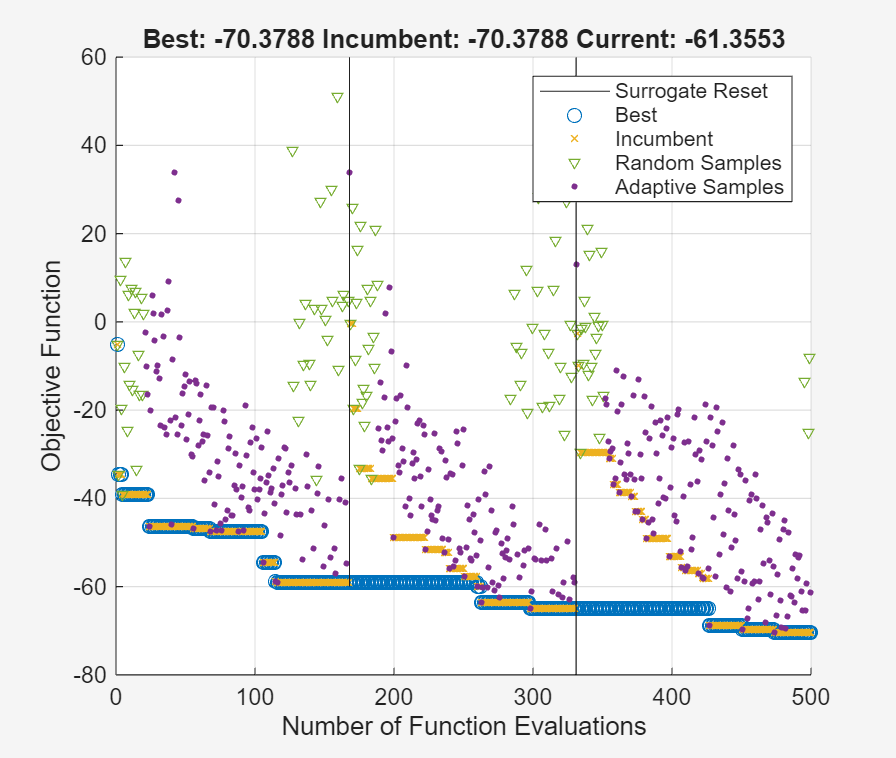```surrogateopt stopped because it exceeded the function evaluation limit set by 'options.MaxFunctionEvaluations'. ```

`surrogateopt` found a point giving an objective function value of –82.5. Investigate the effect of the optimized parameters on the radiation pattern of the antenna.

### Plot Optimized Pattern

Plot the optimized antenna pattern at the design frequency.

```yagidesign.ReflectorLength = optimdesign(1); yagidesign.DirectorLength = optimdesign(2:5); yagidesign.ReflectorSpacing = optimdesign(6); yagidesign.DirectorSpacing = optimdesign(7:10); fig2 = figure; pattern(yagidesign,freq)```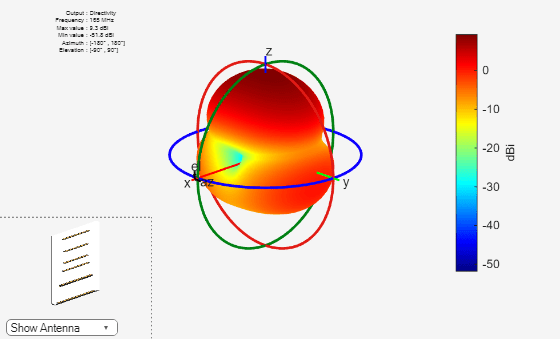Apparently, the antenna now radiates significantly more power at zenith.

### E-Plane and H-Plane Cuts of Pattern

To obtain a better insight into the behavior in two orthogonal planes, plot the normalized magnitude of the electric field in the E-plane and H-plane, that is, azimuth = 0 and 90 deg, respectively.

```fig3 = figure; pattern(yagidesign,freq,0,0:1:359);```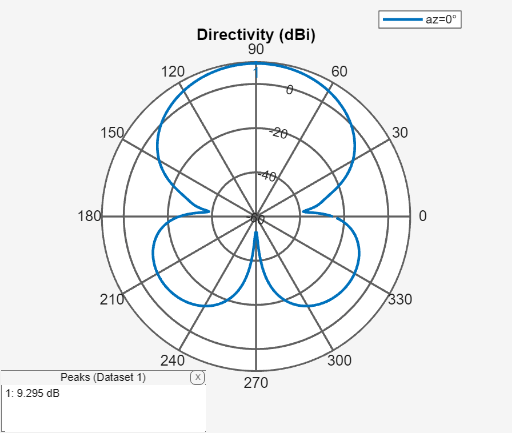```fig4 = figure; pattern(yagidesign,freq,90,0:1:359);```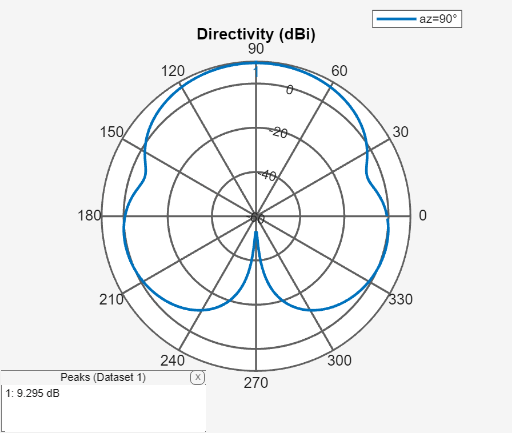The optimized design shows a significant improvement in the radiation pattern. Higher directivity is achieved in the desired direction toward zenith. The back lobe is small, resulting in a good front-to-back ratio for this antenna. Calculate the directivity at zenith, front-to-back ratio, and beamwidth in the E-plane and H-plane.

`D_max = pattern(yagidesign,freq,0,90)`
```D_max = 9.4799 ```
`D_back = pattern(yagidesign,freq,0,-90)`
```D_back = -63.5385 ```
`F_B_ratio = D_max - D_back`
```F_B_ratio = 73.0184 ```
`Eplane_beamwidth = beamwidth(yagidesign,freq,0,1:1:360)`
```Eplane_beamwidth = 56.0000 ```
`Hplane_beamwidth = beamwidth(yagidesign,freq,90,1:1:360)`
```Hplane_beamwidth = 72 ```

### Comparison with Manufacturer Datasheet

The optimized Yagi-Uda antenna achieves a forward directivity of 9.48 dBi, which translates to 7.4 dBd (relative to a dipole). This result is a bit less than the gain value reported by the datasheet in reference  (8.5 dBd). The front-to-back ratio is 73 dB; this is part of the quantity that the optimizer maximizes. The optimized Yagi-Uda antenna has an E-plane beamwidth of 56 deg, which is the same as the datasheet. The H-plane beamwidth of the optimized Yagi-Uda antenna is 72 deg, whereas the value on the datasheet is 63 deg. The example does not address impedance matching over the band.

### Tabulating Initial and Optimized Design

Tabulate the initial design guesses and the final optimized design values.

```yagiparam= {'Reflector Length'; 'Director Length - 1'; 'Director Length - 2'; 'Director Length - 3'; 'Director Length - 4'; 'Reflector Spacing'; 'Director Spacing - 1'; 'Director Spacing - 2';'Director Spacing - 3'; 'Director Spacing - 4'}; initialdesign = initialdesign'; optimdesign = optimdesign'; T = table(initialdesign,optimdesign,RowNames=yagiparam)```
```T=10×2 table initialdesign optimdesign _____________ ___________ Reflector Length 0.90846 1.0009 Director Length - 1 0.90846 0.66951 Director Length - 2 0.90846 0.71935 Director Length - 3 0.90846 0.73777 Director Length - 4 0.90846 0.75073 Reflector Spacing 0.54508 0.32285 Director Spacing - 1 0.45423 0.23736 Director Spacing - 2 0.45423 0.090846 Director Spacing - 3 0.45423 0.37511 Director Spacing - 4 0.45423 0.33403 ```

### Reference

 Balanis, Constantine A. Antenna Theory: Analysis and Design. Fourth edition. Hoboken, New Jersey: Wiley, 2016.